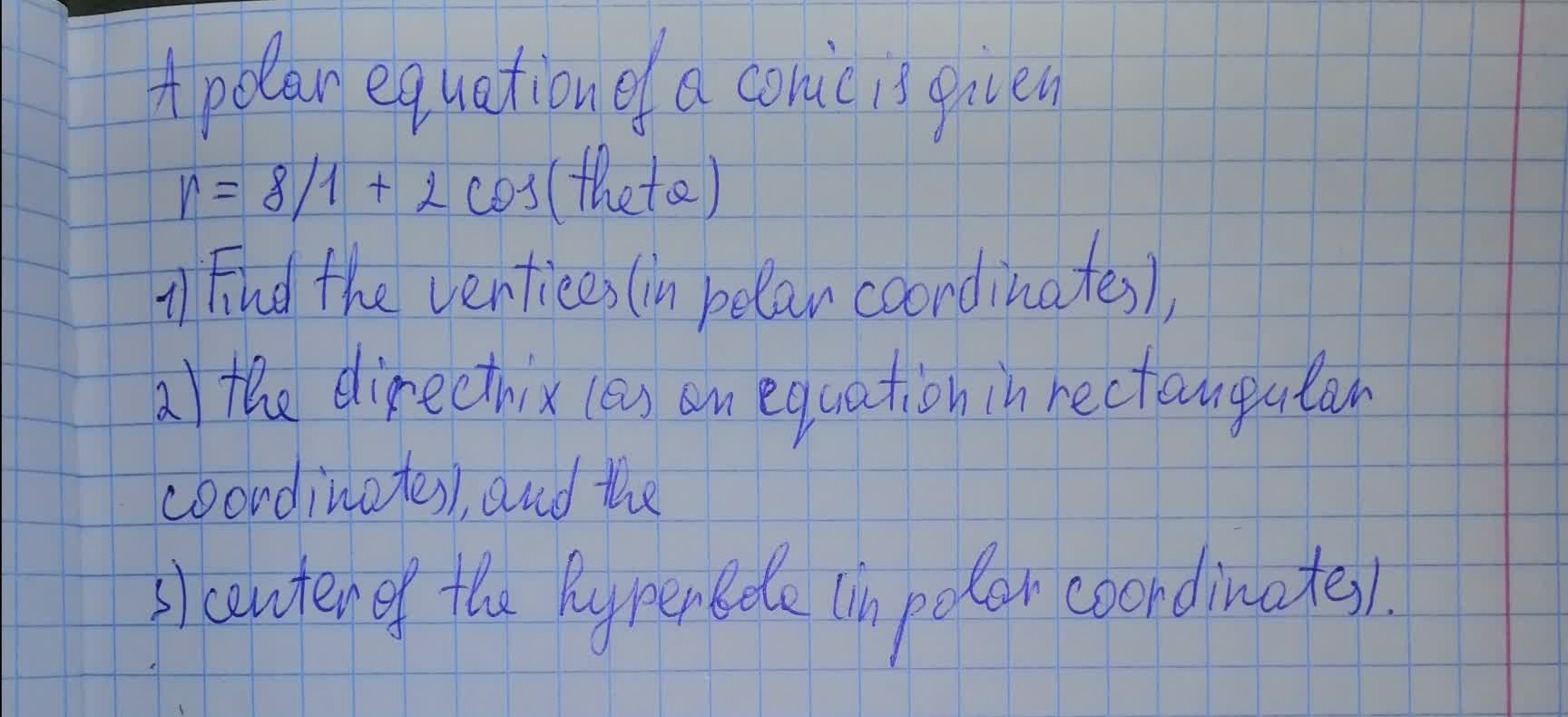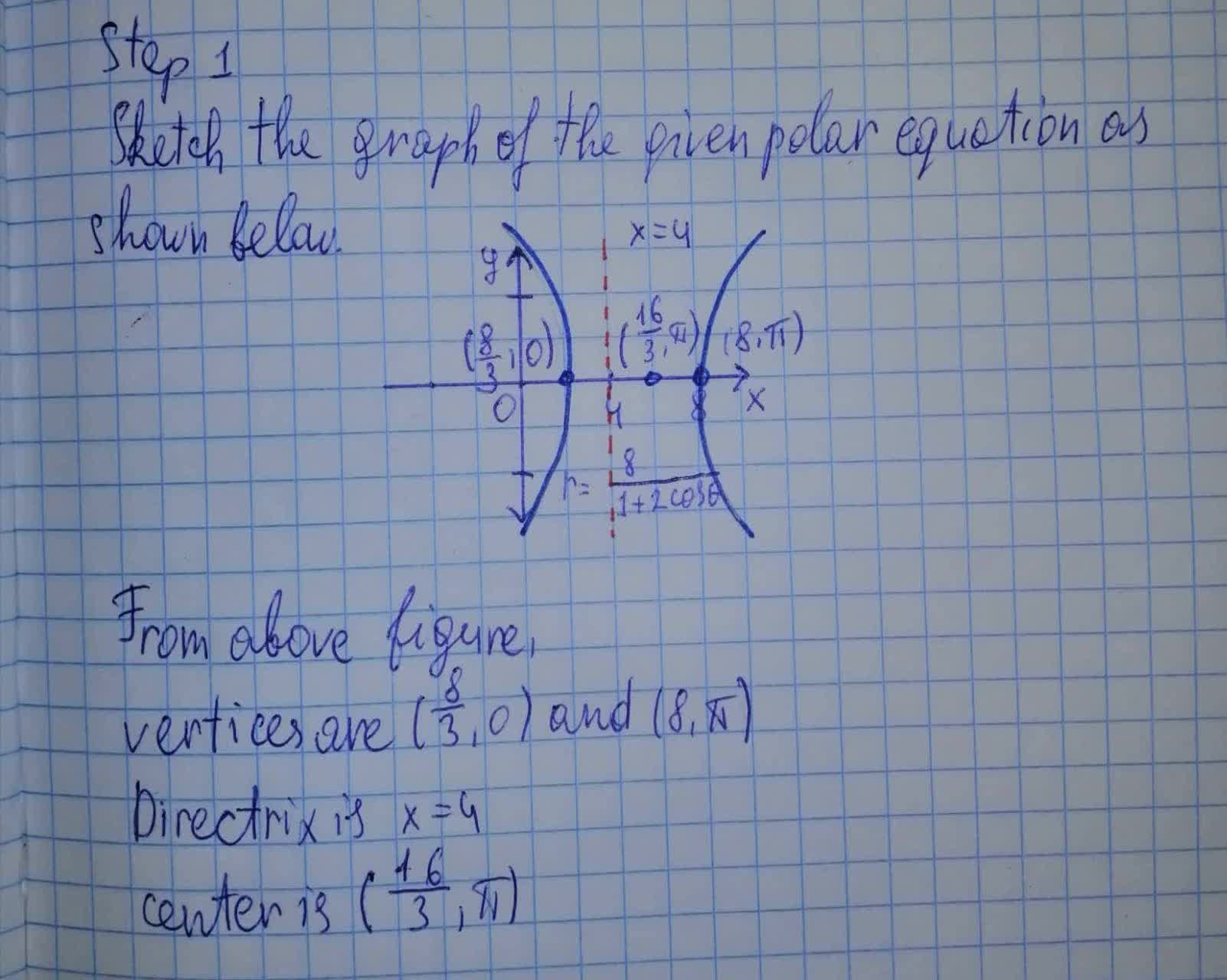Question# A polar equation of a conic is given. r = 8/1 + 2 \cos (\theta)

Conic sections
ANSWEREDA polar equation of a conic is given. $$\displaystyle{r}=\frac{{8}}{{1}}+{2}{\cos{{\left(\theta\right)}}}$$